Weak Law of Large Numbers

Also known as Bernoulli's Theorem. Let, ...,be a sequence of independent and identically distributed random variables, each having a Meanand Standard Deviation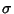. Define a new variable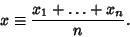(1)

Then, as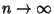, the sample meanequals the population Meanof each variable.(2)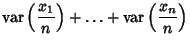(3)

Therefore, by the Chebyshev Inequality, for all,(4)

As, it then follows that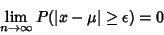(5)

for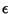arbitrarily small; i.e., as, the sample Mean is the same as the population Mean.

Stated another way, if an event occurstimes inTrials and ifis the probability of success in a single Trial, then the probability that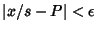foran arbitrary Positive quantity approaches 1 as.

See also Law of Truly Large Numbers, Strong Law of Large Numbers